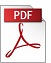### “The partial least squares algorithm: a truncated Cayley-Hamilton series approximation used to solve the regression problem”

Authors: David Di Ruscio,
Affiliation: Telemark University College
Reference: 1998, Vol 19, No 3, pp. 117-140.

Keywords: Least squares, Cayley-Hamilton, PLS

Abstract: In this paper it is shown that the PLS algorithm for univariate data is equivalent to using a truncated Cayley-Hamilton polynomial expression of degree 1 less than a less than r for the matrix inverse inv(X´X) in R^(rxr) used to compute the LS solution. Furthermore, the a coefficients in this polynomial are computed as the LS optimal solution (minimizing parameters) to the prediction error. The resulting solution is non-iterative. The solution can be expressed in terms of one matrix inverse and is given by BPLS = Ka * inv(Ka´*X´*X*Ka)*Ka´*X´*Y where Ka in R^(rxr) is the controllability (Krylov) matrix for the pair (X´X,X´Y).PDF (3422 Kb)        DOI: 10.4173/mic.1998.3.1

References:
 BURNHAM, A.J., VIVEROS, IC MACGREGOR, J.F. (1996). Frameworks for Latent Variable Multivariate Regression, Journal of Chemometrics, Vol. 10, pp. 31-45.
 DE MOOR, B. DAVID, J. (1996). Total least squares and the algebraic Riccati equation, Katholieke Universiteit Leuven, B-3001 Leuven, Belgium. Internal report.
 DI RUSCIO, D. (1997). On subspace identification of the extended observability matrix, In: Proceedings of the 1997 IEEE Conference on Decision and Control, San Diego, California, December 10-12.
 FIERRO, R.D., GOLUB, G.H., HANSEN, P.C. O´LEARY, D.P. (1997). Regularization by truncated total least squares, Siam Journal on Scientific Computing, Vol. 18, No. 4, pp. 1223-1241 doi:10.1137/S1064827594263837
 FRANK, L.E. FRIEDMAN, J.H. (1993). A Statistical View of Some Chemometrics Regression Tool, Technometrics, Vol. 35, No. 2, pp. 109-135 doi:10.2307/1269656
 HANSEN, P.C. (1992). Regularization Tools, A Matlab Package for Analysis and Solution of Discrete Ill-Posed Problems. Danish Computing Centre for Research and Education, DK-2800 Lyngby, Denmark.
 HELLAND, I.S. (1988). On the Structure of Partial Least Squares Regression, Commun. in Stat. Simulation and Computation, Vol. 17, No. 2, pp. 581-607 doi:10.1080/03610918808812681
 HÖSKULDSSON, A. (1996). Prediction Methods in Science and Technology, COLOURSCAN Warsaw, ISBN 87-985941-0-9.
 HÖSKULDSSON, A. (1988). PLS regression methods, Journal of Chemometrics, Vol. 2, pp. 211-228 doi:10.1002/cem.1180020306
 JOHANSEN, T.A. (1997). On Tikhonov Regularization, Bias and Variance, In Nonlinear System Identification. Automatica, Vol. 33, No. 3, pp. 441-446 doi:10.1016/S0005-1098(96)00168-9
 MANNE, R. (1987). Analysis of Two Partial-Least-Squares Algorithms for Multivariate Calibration, Chemometrics and Intelligent Laboratory Systems, Vol. 2, pp. 187-197 doi:10.1016/0169-7439(87)80096-5
 MARTENS, H. NÆS, T. (1989). Multivariate Calibration, John Wiley and Sons Ltd.
 TER BRAAK, C.J. DE JONG, S. (1998). The objective function of Partial Least Squares, Journal of Chemometrics, Vol. 12, pp. 41-54.
 MARTENS, H. NÆS, T. (1985). Comparison of Prediction Methods for Multicolincar Data, Commun. in Stat. Simulation and Computation, Vol. 14, No. 3, pp. 544-576.
 WOLD, H. (1966). Non-linear estimation by iterative least squares procedures, Research papers in statistics, Ed. F. David. Wiley, New York, pp. 411-444.
 WOLD, H. (1975). Soft modeling by latent variables: the Non-linear Iterative Partial Least Squares Approach, In: Perspectives in Probability and Statistics, Editor J. Gani. London, Academic Press.
 WOLD, H. (1985). Partial Least Squares, In: Encyclopedia of statistics sciences, Editors S. Kotz and N.L. Johnson. Wiley, Vol. 6, pp. 581-591.
 LORBER, A., LAWRENCE, E.W. KOWALSKI B.R. (1987). A Theoretical Foundation for the PLS Algorithm, Journal of Chemometrics, Vol. 1, pp. 19-31 doi:10.1002/cem.1180010105
 VETTER, W.J. (1973). Matrix calculus operations and Taylor expansions, Siam review, Vol. 15, No. 2, pp. 352-369 doi:10.1137/1015034

BibTeX:
@article{MIC-1998-3-1,
title={{The partial least squares algorithm: a truncated Cayley-Hamilton series approximation used to solve the regression problem}},
author={Di Ruscio, David},
journal={Modeling, Identification and Control},
volume={19},
number={3},
pages={117--140},
year={1998},
doi={10.4173/mic.1998.3.1},
publisher={Norwegian Society of Automatic Control}
};